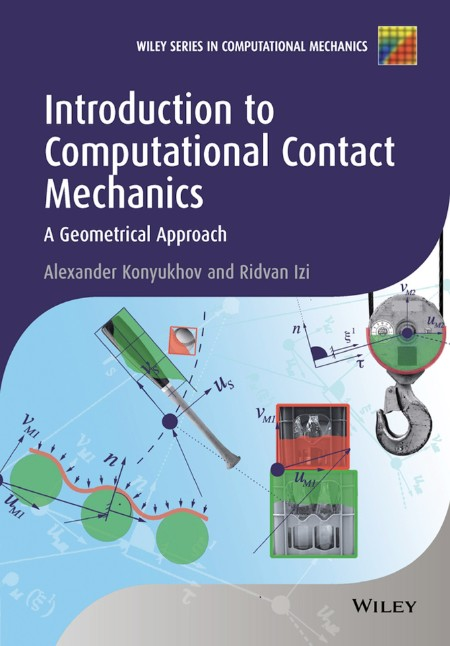# Konyukhov A Introduction to Computational Contact Mechanics Geometrical 2015

Posted in: eBooks | By: AD-TEAM | 7-09-2023, 21:32 | 0 Comments
07
September
2023pdf | 7.53 MB | N/A | Isbn:1118770633 | Author: Alexander Konyukhov | Year: 2014

Description:

Introduction to Computational Contact Mechanics: A Geometrical Approach covers the fundamentals of computational contact mechanics and focuses on its practical implementation. Part one of this textbook focuses on the underlying theory and covers essential information about differential geometry and mathematical methods which are necessary to build the computational algorithm independently from other courses in mechanics. The geometrically exact theory for the computational contact mechanics is described in step-by-step manner, using examples of strict derivation from a mathematical point of view. The final goal of the theory is to construct in the independent approximation form /so-called covariant form, including application to high-order and isogeometric finite elements.

The second part of a book is a practical guide for programming of contact elements and is written in such a way that makes it easy for a programmer to implement using any programming language. All programming examples are accompanied by a set of verification examples allowing the user to learn the research verification technique, essential for the computational contact analysis.

Key features:

Covers the fundamentals of computational contact mechanics Covers practical programming, verification and analysis of contact problems Presents the geometrically exact theory for computational contact mechanics Describes algorithms used in well-known finite element software packages Describes modeling of forces as an inverse contact algorithm Includes practical exercises Contains unique verification examples such as the generalized Euler formula for a rope on a surface, and the impact problem and verification of th� percussion center Accompanied by a website hosting software Introduction to Computational Contact Mechanics: A Geometrical Approach is an ideal textbook for graduates and senior undergraduates, and is also a useful reference for researchers and practitioners working in computational mechanics.